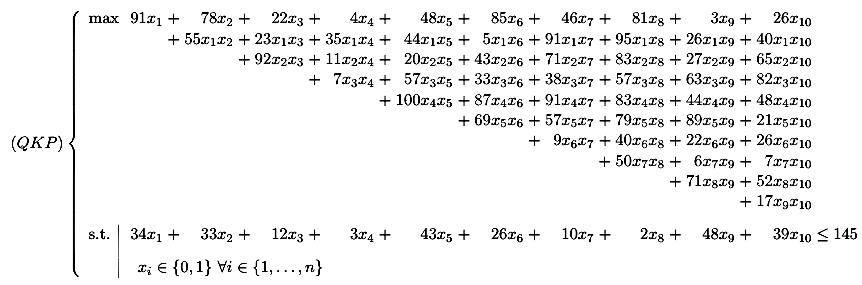(QKP) Instances

Back to (QKP) Instances main page

Each (QKP) instance correspond to a file containing the following informations:

• the reference of the instance (r_10_100_13 in the following example)
• the number of variables (n) (10 in the following example)
• the linear coefficients (c_i) of the objective function (91 78 22 4 48 85 46 81 3 26)
• the quadratic coefficients (c_ij) of the objective function
• a blank line
• 0 if the constraint is of type <= (i.e. always since we are considering (QKP) instances) and 1 if the constraint is an eglity constraint
• the capacity of the knapsack (145)
• the coefficients of the capacity constraints (weights, a_i) (34 33 12 3 43 26 10 2 48 39 )

Here is an example concerning a 10-variable instance:

r_10_100_13
10
91 78 22 4 48 85 46 81 3 26
55 23 35 44 5 91 95 26 40
92 11 20 43 71 83 27 65
7 57 33 38 57 63 82
100 87 91 83 44 48
69 57 79 89 21
9 40 22 26
50 6 7
71 52
17

0
145
34 33 12 3 43 26 10 2 48 39